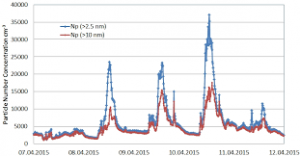## How to Calculate and Solve for the Volume of a Constituent, Volume of a Mixture and Volume Concentration in Chemistry | The Calculator EncyclopediaThe image above represents the volume concentration.

To compute the volume concentration of a substance, two essential parameters are needed and these parameters are volume of the constituent (Vi) and volume of the mixture (V).

The formula for calculating volume concentration:

φ = ViV

Where;
φ = Volume concentration
Vi = Volume of the constituent
V = Volume of the mixture

Let’s solve an example;
Find the volume concentration with a volume of the constituent of 23 and a volume of the mixture of 32.

This implies that;
Vi = Volume of the constituent = 23
V = Volume of the mixture = 32

φ = ViV
φ = 2332
φ = 0.718

Therefore, the volume concentration is 0.718.

Calculating the Volume of the Constituent when the Volume Concentration and the Volume of the Mixture is Given.

Vi = Vφ

Where;
φ = Volume concentration
V = Volume of the mixture

Let’s solve an example;
Find the volume of the constituent with a volume concentration of 18 and a volume of the mixture of 22.

This implies that;
φ = Volume concentration = 18
V = Volume of the mixture = 22

Vi = Vφ
Vi = 18 x 22
Vi = 396

Therefore, the volume of the constituent is 396 cm3.

## How to Calculate and Solve for the Number of Entities of a Constituent, Volume and Number Concentration in Chemistry | Nickzom CalculatorThe image above represents the number concentration.

To compute for the number concentration, two essential parameters are needed and these parameters are Number of Entities of the Constituent (N) and Volume (V).

The formula for calculating the number concentration:

C = NV

Where;
C = Number Concentration
N = Number of Entities of the Constituent
V = Volume

Let’s solve an example;
Find the number concentration when the number of entities of the constituent is 12 and the volume is 24.

This implies that;
N = Number of Entities of the Constituent = 12
V = Volume = 24

C = NV
C = 1224
C = 0.5

Therefore, the number concentration is 0.5 m-3.

Calculating the Number of Entities of the Constituent when Number Concentration and Volume is Given.

N = VC

Where;
N = Number of Entities of the Constituent
C = Number Concentration
V = Volume

Let’s solve an example;
Find the number of entities of the constituent when the number concentration is 18 and the volume is 14.

This implies that;
C = Number Concentration = 18
V = Volume = 14

N = VC
N = 14 x 18
N = 252

Therefore, the number of entities of the constituent is 252 m-1.